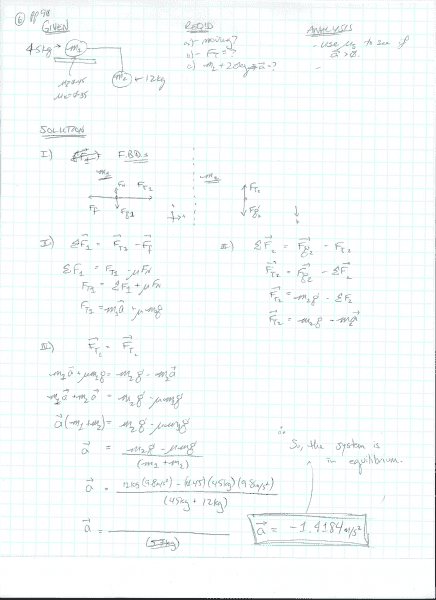# Interpret results of suspended mass / tension problem please

• Tyrannosaurus_
In summary, the homework statement states that two masses, each 45kg, are sitting on a table top with static friction co. 0.45, kinetic friction co. 0.35. m1 is attached to m2 by a massless wire hung over a frictionless pulley. The attempt at a solution finds that m1 is at rest and that the negative acceleration value arises from assuming the frictional force equals μsN.

## Homework Statement

Two masses: m1 = 45 kg, m2 = 12kg.
m1 (45kg) sits at rest on a table top with static friction co. 0.45, kinetic friction co. 0.35.
m2 is suspended in the air. m2 is attached to m1 by a massless wire hung over a frictionless pulley

## Homework Equations

FBDs, net force equations

## The Attempt at a Solution

m1 is at rest, so I'll use the μs = 0.45.
I've attached an image of my solution.

My question is how do I interpret the negative acceleration value? I know it's a vector, but it's not as though the simple mass that is sitting on the table can accelerate, and even pull the 12kg mass even further higher into the air. So, does this mean that the system is at rest and there would be no acceleration because Fg is not greater than the Fk?

Many thanks!Tyrannosaurus_ said:
m1 is at rest, so I'll use the μs = 0.45.
That only makes sense as far as determining whether the system will remain at rest. If not, you need to switch to kinetic friction to discover the acceleration.
Your working is hard to read. You should only post images for diagrams and textbook extracts. Please take the trouble to type other working in directly. Not only is it easier to read, it's easier to identify sections of working when posting replies.
Tyrannosaurus_ said:
how do I interpret the negative acceleration value?
That probably arises from assuming the frictional force equals μsN. μsN is merely the maximum possible frictional force. In equilibrium, it will usually be less than that.

•Tyrannosaurus_
It's too difficult to follow your work, but I think I can deduce the error you made from your answer. You treated m1s as if it were the actual force of friction acting. This is not correct; m1s represents the maximum force that static friction is capable of exerting. The actual force of static friction will only be as much it needs to be to keep the block at rest (unless of course m1s is not large enough, in which case the system will accelerate and only µk will be relevant).

Thanks for the help! Much appreciated!